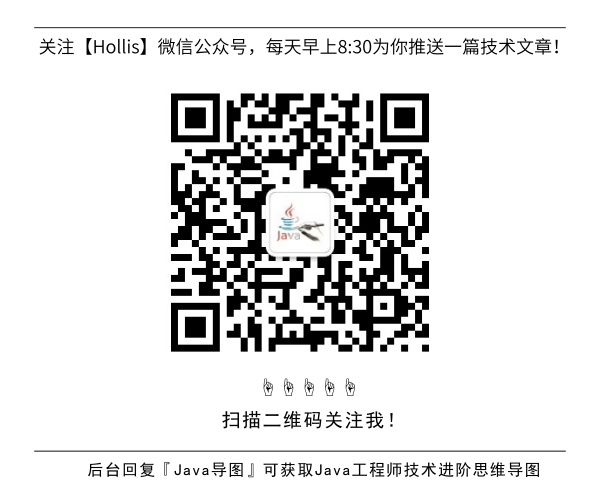# 使用两个栈实现队列，使用两个队列实现栈。

GitHub 19k Star 的Java工程师成神之路，不来了解一下吗！

### 栈的队列的特点

• isEmpty()
• 判断栈是否为空
• size()
• 返回栈中元素的个数
• push()
• 向栈中插入数据
• pop()
• 从栈中弹出数据并返回
• peek()
• 返回栈顶的数据

• isEmpty()
• 判断队列是否为空
• size()
• 返回队列中元素的个数
• enqueue()
• 向队列中插入数据
• dequeue()
• 从队列中弹出数据并返回
• peek()
• 返回队列中第一个数据

### 使用栈来实现队列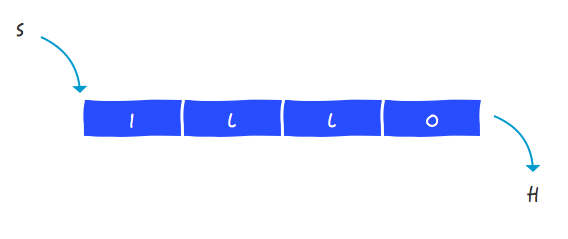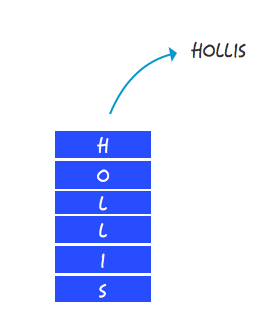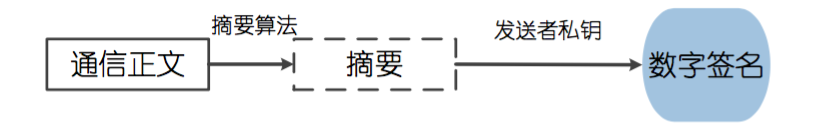``````class MyQueue {
stack<int> in;
stack<int> out;
}
``````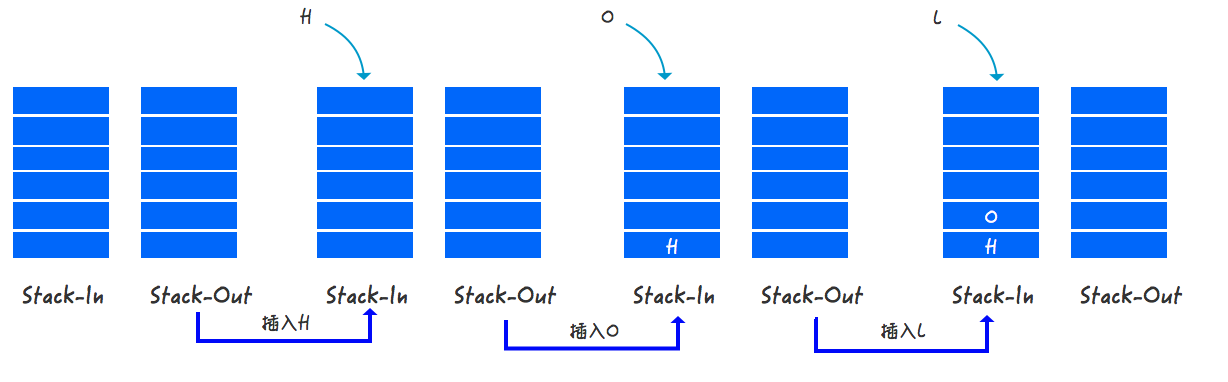``````void push(int x) {
in.push(x);
}
``````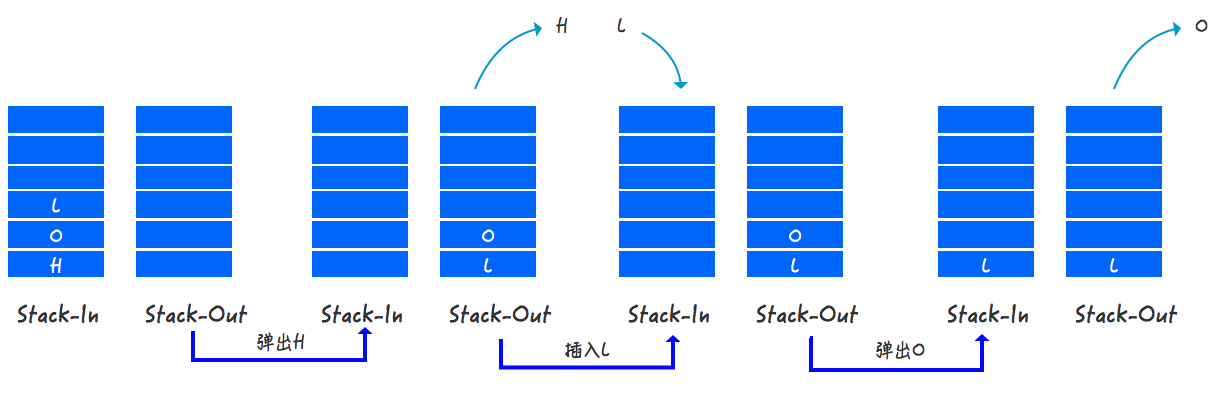``````int pop() {
if (!out.empty()) {
int temp = out.top();
out.pop();
return temp;
}
else {
if (in.empty()) {
return -1;
}
else {
while (!in.empty()) {
out.push(in.top());
in.pop();
}
int temp = out.top();
out.pop();
return temp;
}
}
}
``````

### 使用队列来实现栈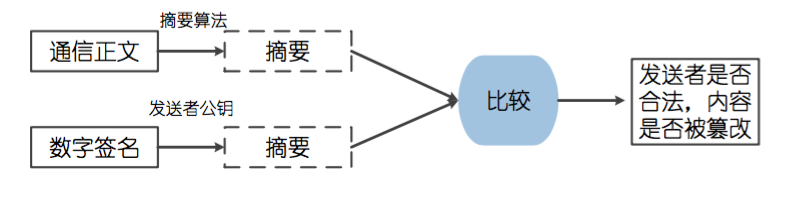``````class MyStack {
queue<int> a;
queue<int> b;
}
``````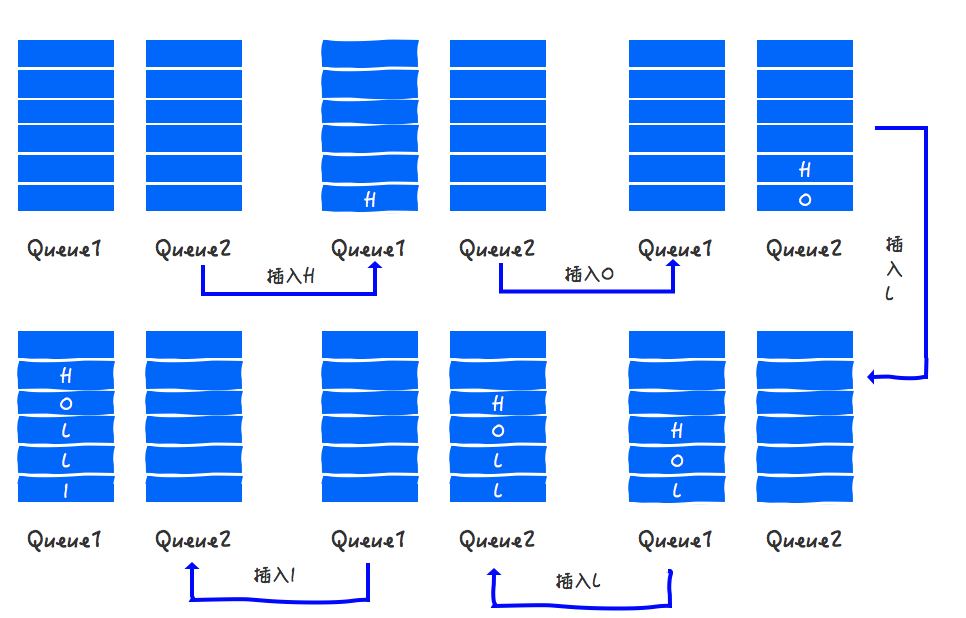``````void push(int x) {
if (a.empty() && b.empty()) {
a.push(x);
return;
}
if (a.empty()) {
a.push(x);
while (!b.empty()) {
a.push(b.front());
b.pop();
}
}
else {
b.push(x);
while (!a.empty()) {
b.push(a.front());
a.pop();
}
}
}
``````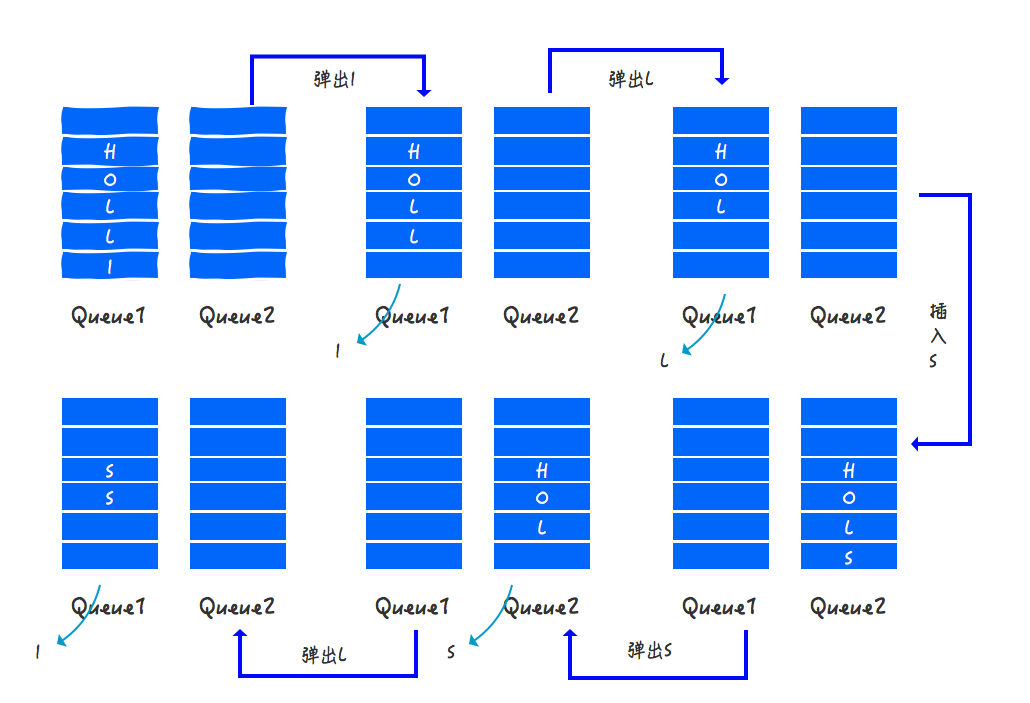``````int pop() {
if (a.empty()) {
int temp = b.front();
b.pop();
return temp;
}
else {
int temp = a.front();
a.pop();
return temp;
}
}
``````(全文完)### 评论 1

1. #1

真的很棒！希望能有更多更丰富的文章，帮助小白程序猿理解计算机语言！！

青崖3年前 (2019-10-30)回复

## HollisChuang's Blog

• 微信咨询
• 去评论
• 回顶
###### 回顶部